# Convert State Name to Abbreviation – Excel & Google Sheets

This tutorial will demonstrate how convert a state name to an abbreviation in Excel and Google Sheets.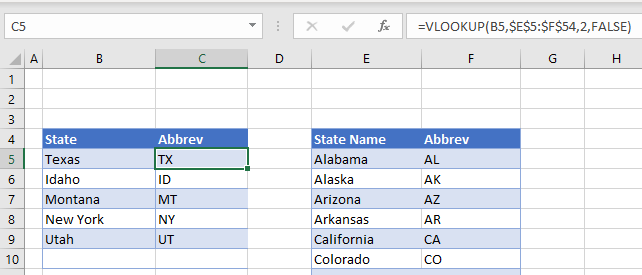To convert a state name to an abbreviation, we can use the VLOOKUP Function.

## VLOOKUP Function

The VLOOKUP Function searches for a value in the leftmost column of a table and then returns a value a specified number of columns to the right from the found value.

First, we need to create a table with the lookup values in it.  The state name would be in the first column, and the abbreviation in the second column.Although the graphic above is only showing the first 13 states, the lookup table would need to have all 50 states in it.

Next, we use a VLOOKUP function to look up the State abbreviation.

``=VLOOKUP(B5,\$E\$5:\$F\$54,2,FALSE)``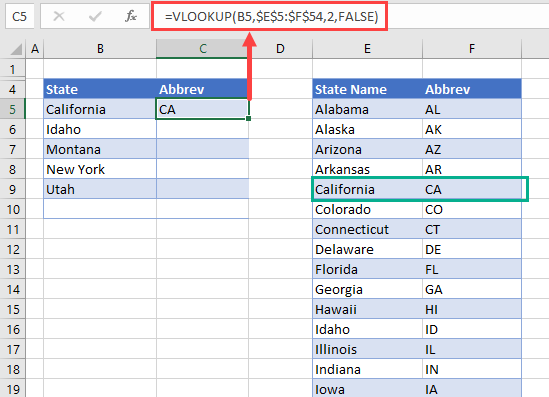Because we have used an absolute for the lookup table (\$), we can then copy the formula down to the other rows of our table.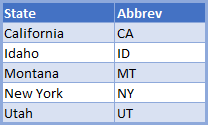### Using a RANGE Name

If you do not want to highlight the lookup table within your formula, before you create the formula, you can create a range name for the lookup table.

Highlight the range eg: E5:F54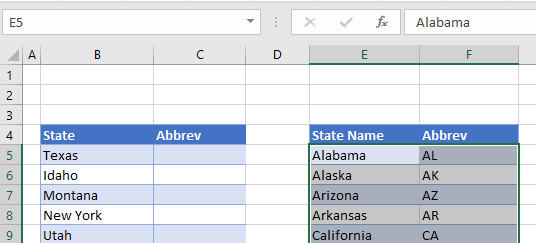Click in the names box and type the word STATES and then press the Enter key on the keyboard.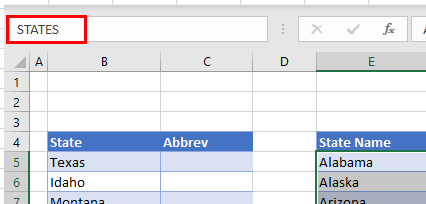You will now be able to select the named range from the drop down list in the name box.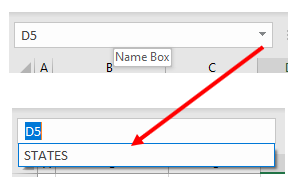This will highlight all the cells in the names range.

You can now use this range name in your VLOOKUP formula instead of using the actual range of cells.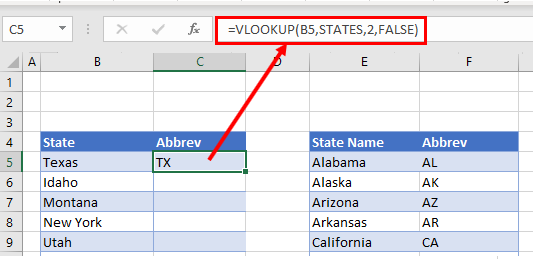## Convert State Name to Abbreviation in Google Sheets

The VLOOKUP function works the same way in Google Sheets as it does in Excel.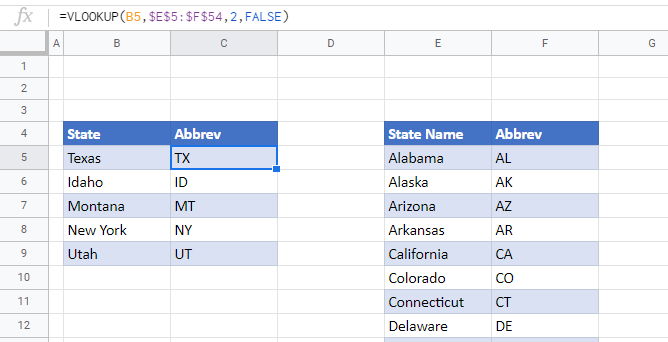### Excel Practice Worksheet

Practice Excel functions and formulas with our 100% free practice worksheets!